# Selina Solutions Concise Maths Class 10 Chapter 5 Quadratic Equations Exercise 5(E)

Some equations may not look like a quadratic equation but can be reducible to quadratic equations. This reduction is done by proper substitution. Thus, in this exercise students will be solving different kinds of quadratic equations. For quick reference to any problem, the Selina Solutions for Class 10 Maths can be used. The solutions of this exercise are available in the Concise Selina Solutions for Class 10 Maths Chapter 5 Quadratic Equations Exercise 5(E), PDF provided in the links below.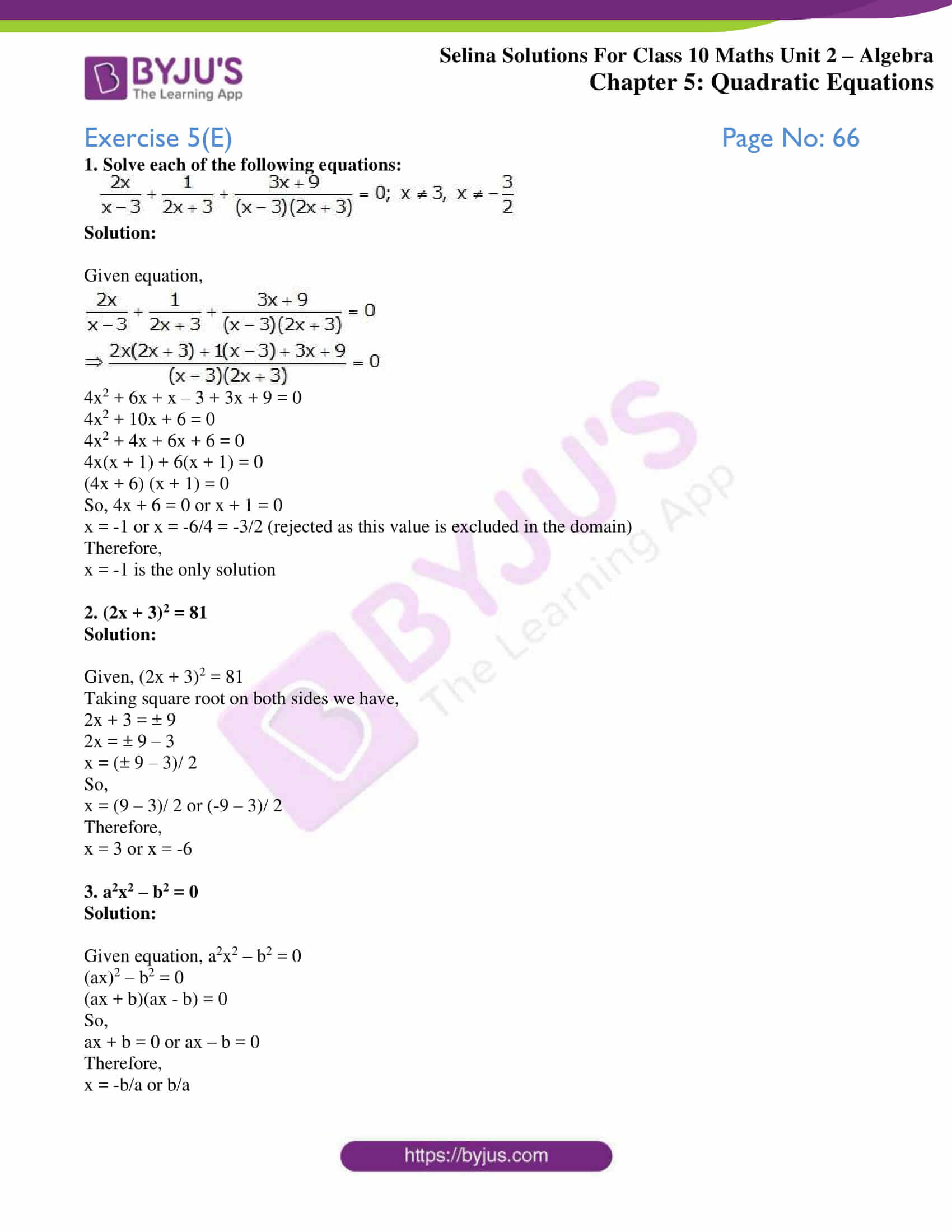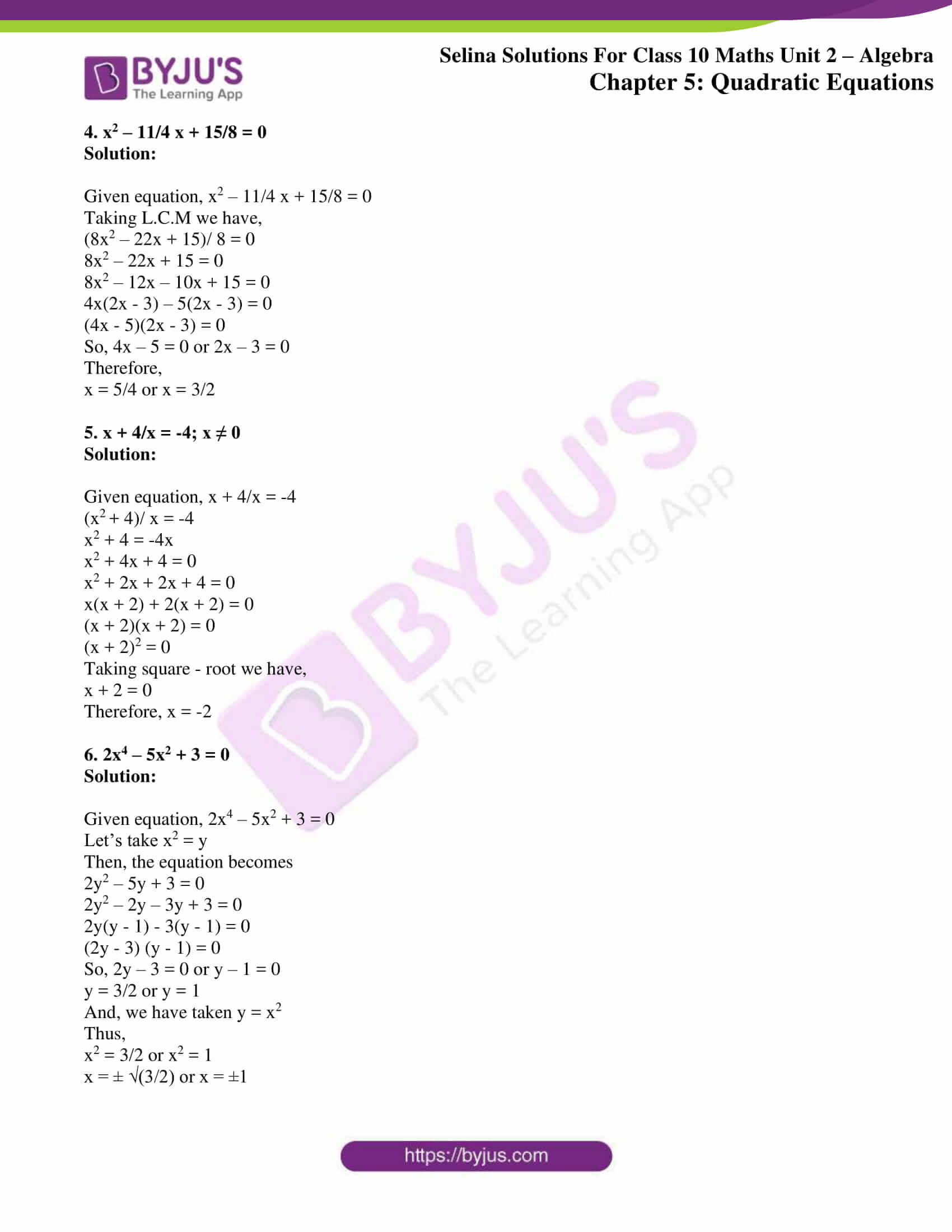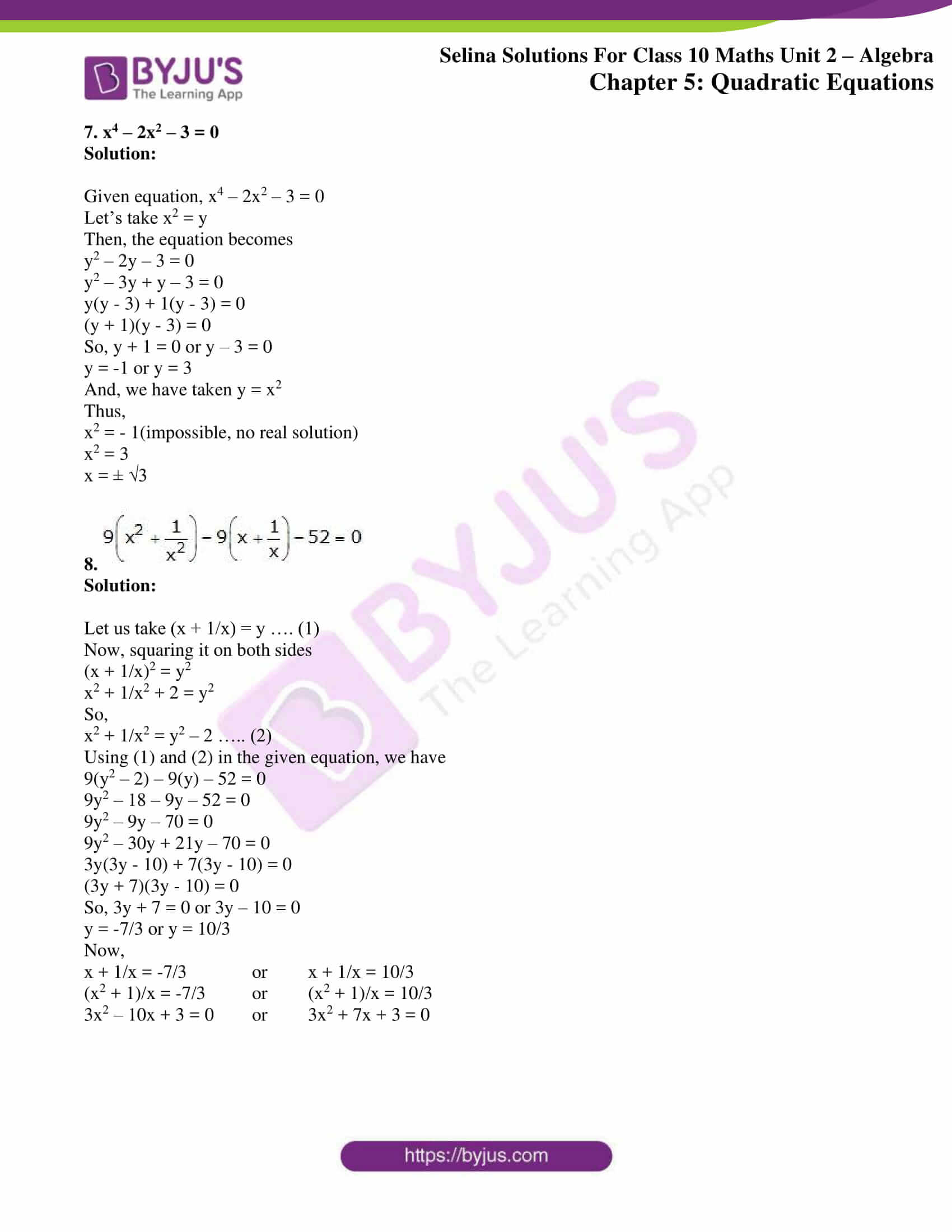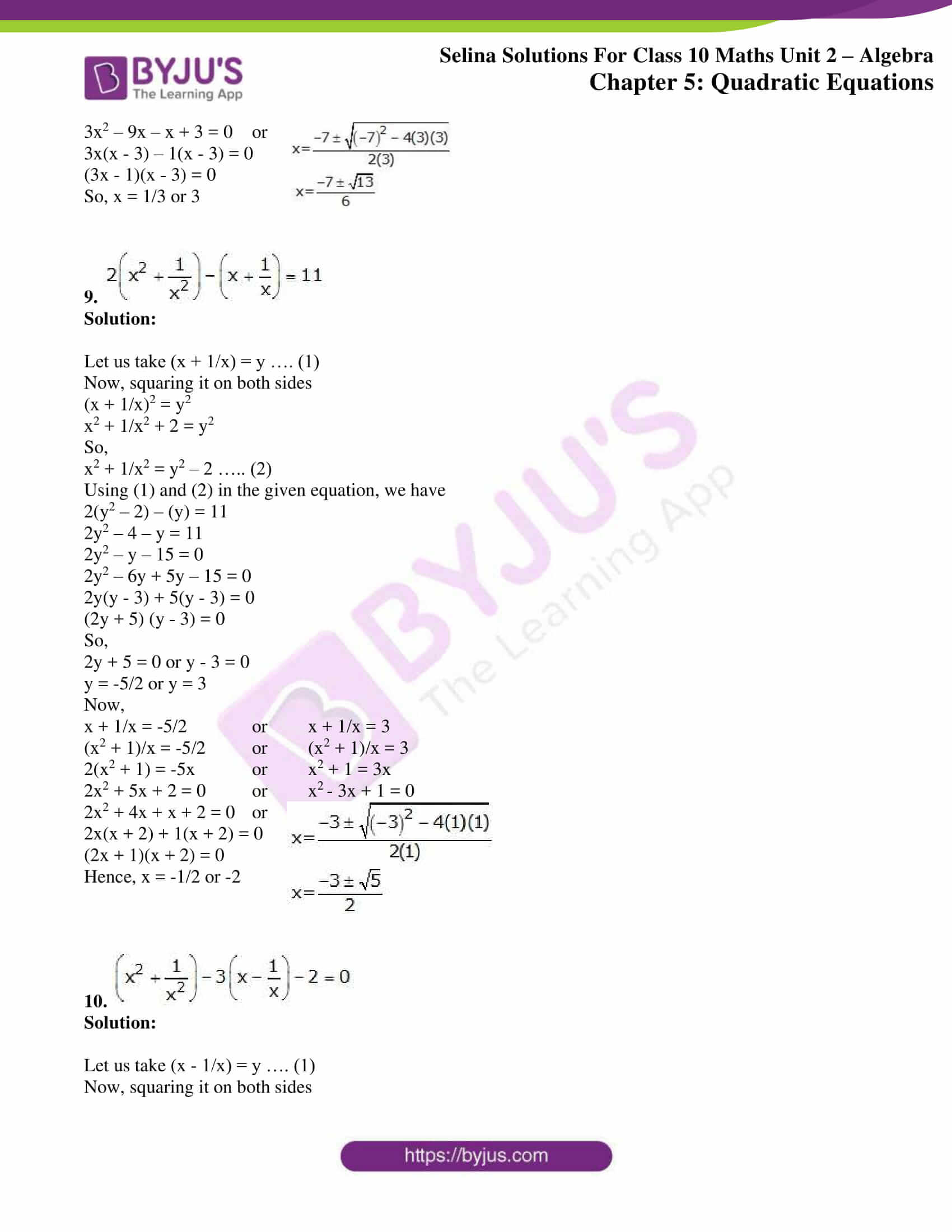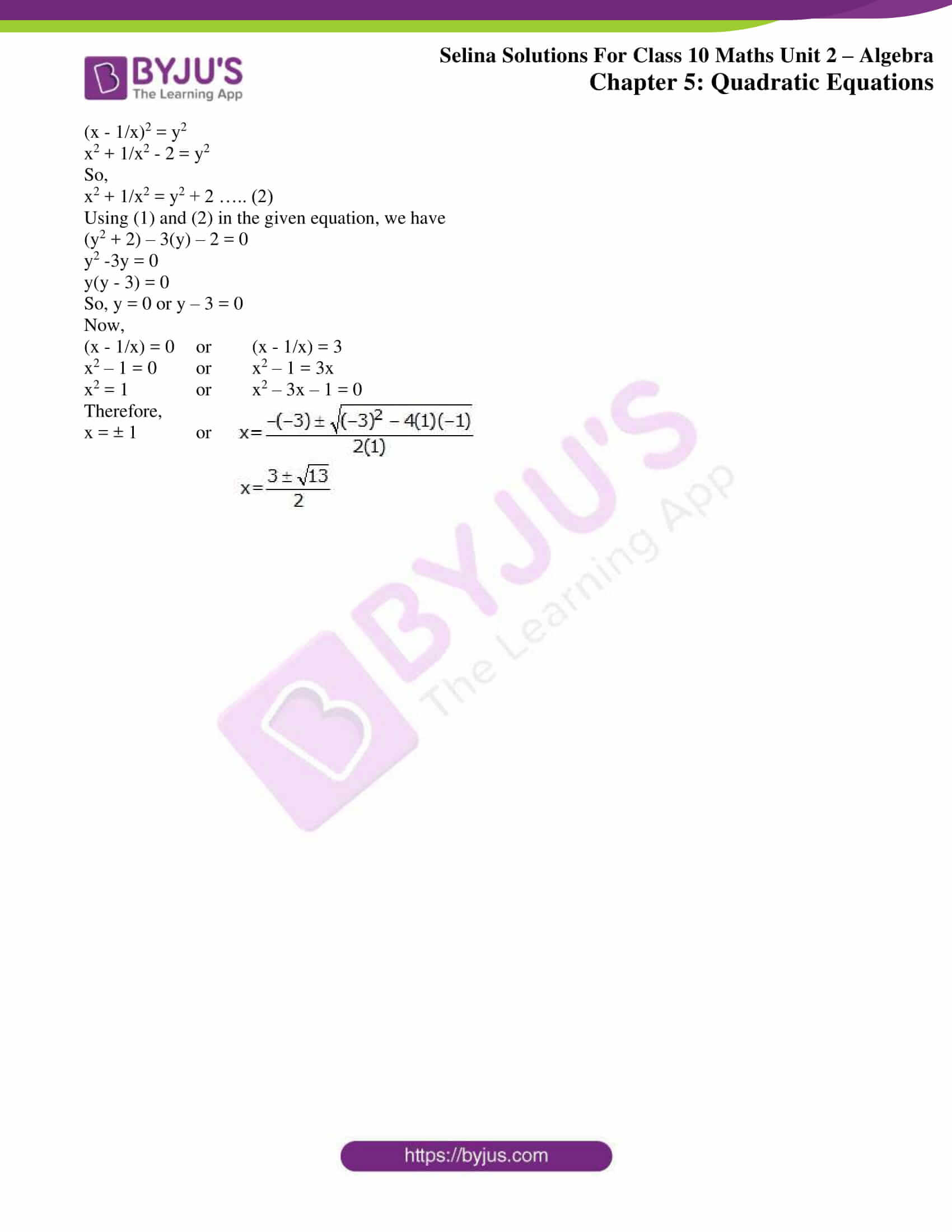### Access Selina Solutions Concise Maths Class 10 Chapter 5 Quadratic Equations Exercise 5(E)

1. Solve each of the following equations: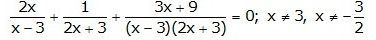Solution:

Given equation,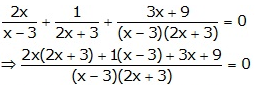4x2 + 6x + x – 3 + 3x + 9 = 0

4x2 + 10x + 6 = 0

4x2 + 4x + 6x + 6 = 0

4x(x + 1) + 6(x + 1) = 0

(4x + 6) (x + 1) = 0

So, 4x + 6 = 0 or x + 1 = 0

x = -1 or x = -6/4 = -3/2 (rejected as this value is excluded in the domain)

Therefore,

x = -1 is the only solution

2. (2x + 3)2 = 81

Solution:

Given, (2x + 3)2 = 81

Taking square root on both sides we have,

2x + 3 = ± 9

2x = ± 9 – 3

x = (± 9 – 3)/ 2

So,

x = (9 – 3)/ 2 or (-9 – 3)/ 2

Therefore,

x = 3 or x = -6

3. a2x2 – b2 = 0

Solution:

Given equation, a2x2 – b2 = 0

(ax)2 – b2 = 0

(ax + b)(ax – b) = 0

So,

ax + b = 0 or ax – b = 0

Therefore,

x = -b/a or b/a

4. x2 – 11/4 x + 15/8 = 0

Solution:

Given equation, x2 – 11/4 x + 15/8 = 0

Taking L.C.M we have,

(8x2 – 22x + 15)/ 8 = 0

8x2 – 22x + 15 = 0

8x2 – 12x – 10x + 15 = 0

4x(2x – 3) – 5(2x – 3) = 0

(4x – 5)(2x – 3) = 0

So, 4x – 5 = 0 or 2x – 3 = 0

Therefore,

x = 5/4 or x = 3/2

5. x + 4/x = -4; x ≠ 0

Solution:

Given equation, x + 4/x = -4

(x2 + 4)/ x = -4

x2 + 4 = -4x

x2 + 4x + 4 = 0

x2 + 2x + 2x + 4 = 0

x(x + 2) + 2(x + 2) = 0

(x + 2)(x + 2) = 0

(x + 2)2 = 0

Taking square – root we have,

x + 2 = 0

Therefore, x = -2

6. 2x4 – 5x2 + 3 = 0

Solution:

Given equation, 2x4 – 5x2 + 3 = 0

Let’s take x2 = y

Then, the equation becomes

2y2 – 5y + 3 = 0

2y2 – 2y – 3y + 3 = 0

2y(y – 1) – 3(y – 1) = 0

(2y – 3) (y – 1) = 0

So, 2y – 3 = 0 or y – 1 = 0

y = 3/2 or y = 1

And, we have taken y = x2

Thus,

x2 = 3/2 or x2 = 1

x = ± √(3/2) or x = ±1

7. x4 – 2x2 – 3 = 0

Solution:

Given equation, x4 – 2x2 – 3 = 0

Let’s take x2 = y

Then, the equation becomes

y2 – 2y – 3 = 0

y2 – 3y + y – 3 = 0

y(y – 3) + 1(y – 3) = 0

(y + 1)(y – 3) = 0

So, y + 1 = 0 or y – 3 = 0

y = -1 or y = 3

And, we have taken y = x2

Thus,

x2 = – 1(impossible, no real solution)

x2 = 3

x = ± √3

8.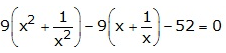Solution:

Let us take (x + 1/x) = y …. (1)

Now, squaring it on both sides

(x + 1/x)2 = y2

x2 + 1/x2 + 2 = y2

So,

x2 + 1/x2 = y2 – 2 ….. (2)

Using (1) and (2) in the given equation, we have

9(y2 – 2) – 9(y) – 52 = 0

9y2 – 18 – 9y – 52 = 0

9y2 – 9y – 70 = 0

9y2 – 30y + 21y – 70 = 0

3y(3y – 10) + 7(3y – 10) = 0

(3y + 7)(3y – 10) = 0

So, 3y + 7 = 0 or 3y – 10 = 0

y = -7/3 or y = 10/3

Now,

x + 1/x = -7/3 or x + 1/x = 10/3

(x2 + 1)/x = -7/3 or (x2 + 1)/x = 10/3

3x2 – 10x + 3 = 0 or 3x2 + 7x + 3 = 0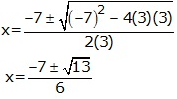3x2 – 9x – x + 3 = 0 or

3x(x – 3) – 1(x – 3) = 0

(3x – 1)(x – 3) = 0

So, x = 1/3 or 3

9.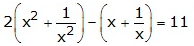Solution:

Let us take (x + 1/x) = y …. (1)

Now, squaring it on both sides

(x + 1/x)2 = y2

x2 + 1/x2 + 2 = y2

So,

x2 + 1/x2 = y2 – 2 ….. (2)

Using (1) and (2) in the given equation, we have

2(y2 – 2) – (y) = 11

2y2 – 4 – y = 11

2y2 – y – 15 = 0

2y2 – 6y + 5y – 15 = 0

2y(y – 3) + 5(y – 3) = 0

(2y + 5) (y – 3) = 0

So,

2y + 5 = 0 or y – 3 = 0

y = -5/2 or y = 3

Now,

x + 1/x = -5/2 or x + 1/x = 3

(x2 + 1)/x = -5/2 or (x2 + 1)/x = 3

2(x2 + 1) = -5x or x2 + 1 = 3x

2x2 + 5x + 2 = 0 or x2 – 3x + 1 = 0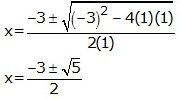2x2 + 4x + x + 2 = 0 or

2x(x + 2) + 1(x + 2) = 0

(2x + 1)(x + 2) = 0

Hence, x = -1/2 or -2

10.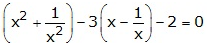Solution:

Let us take (x – 1/x) = y …. (1)

Now, squaring it on both sides

(x – 1/x)2 = y2

x2 + 1/x2 – 2 = y2

So,

x2 + 1/x2 = y2 + 2 ….. (2)

Using (1) and (2) in the given equation, we have

(y2 + 2) – 3(y) – 2 = 0

y2 -3y = 0

y(y – 3) = 0

So, y = 0 or y – 3 = 0

Now,

(x – 1/x) = 0 or (x – 1/x) = 3

x2 – 1 = 0 or x2 – 1 = 3x

x2 = 1 or x2 – 3x – 1 = 0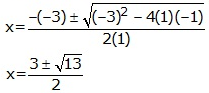Therefore,

x = ± 1 or